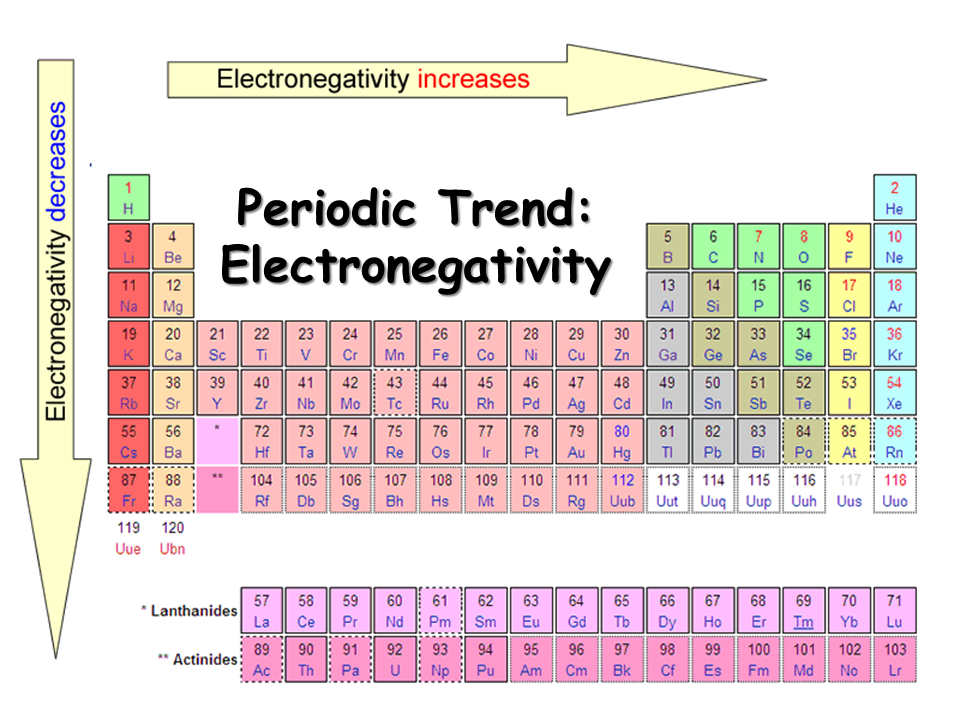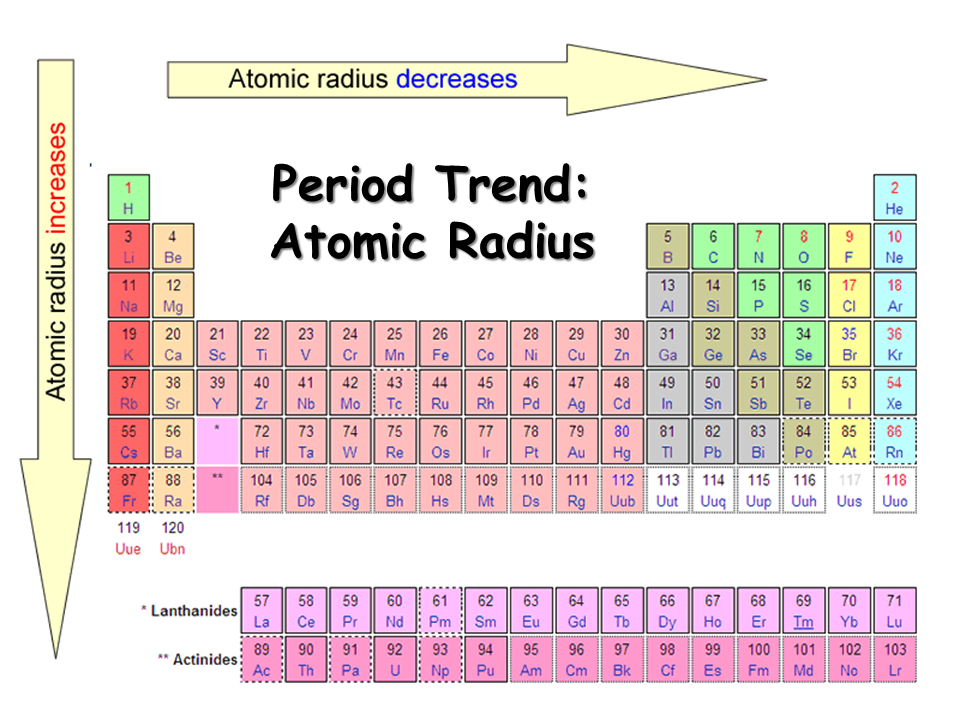Resources:

Have you found videos, websites, or explanations that helped you understand this chapter? Let us know and we'll add them to "Resources" part of this page for other students to use.

Question 1

Which of these elements is predicted to be the most active metal?Select one:Explanation

The element with smallest electronegativity is most active because it is located group 1A (most reactive metals)Question 2

Which of these elements is predicted to be the most active nonmetal?Select one:Explanation

The element with largest electronegativity is the most reactive nonmetal.Question 3

When C30H50O2 is completely oxidized with excess oxygen, what are the products?

Select one:Explanation

This is a combustion reaction so the products are always going to H2O and CO2.

Question 4

In what part of the periodic table are the largest electronegativities found?

Select one:Explanation

electronegativity - the ability of an atom to gain an electronQuestion 5

In what part of the periodic table are the smallest ionization energies for the first electron found?

Select one:ExplanationIonization - the energy required to lose an electron

Question 6

Arrange the elements Li, Be, Ne, and Ar in increasing order of the energy required to remove an electron from their respective gaseous atoms
.
Select one:Explanation

Ionization - the energy required to lose an electronQuestion 7

The first four ionization energies of an element X are 0.58, 1.82, 2.74, and 11.58 MJ/mol. What is the most likely formula for a stable ion of X?
Select one:Explanation

After removing 3 valence electrons the the fourth ionization energy is really large due to the removal of a core electron. Therefore, the stable form is X3+

Question 8

Which statement is correct concerning noble gases?

Select one:Explanation

Noble gases are normally in a gas state at room temperature. Other elements become negative or positive charged to become like noble gases. Noble gases were once inert.

Question 9

If the formula of an oxide of element X is XO, what is the formula of the chloride of X?

Select one:Explanation

Oxyen is -2, which means X is +2.

Cl : -1

Cross the charges to get:Question 10

If the formula of an oxide of element X is XO, what is the formula of the nitride of X?

Select one:Explanation

Oxyen is -2, which means X is +2.

N : -3

Cross the charges to get: X3N2

Question 11

If silicon, atomic number 14, combines with chlorine, atomic number 17 what is the most likely formula based on your knowledge of the periodic nature of the elements?

Select one:Explanation

Si : -4

Cl: -1

Cross the charges to get :  SiCl4

Question 12

When the three elements P, Cl, and As are arranged in order of increasing atomic radius, which is the correct order?

Select one:ExplanationQuestion 13

When the three elements Na, Al, and K are arranged in order of increasing atomic radius, which is the correct order?

Select one:ExplanationQuestion 14

When the three ions N3-, O2-, and F- are arranged in order of increasing ionic radius, which is the correct order?

Select one:Explanation

 protons electrons size F- 9 10 small (strong  attraction to nucleus) O2- 8 10 medium (medium attraction to nucleus) N3- 7 10 large ( weak attraction to nucleus)

Question 15

When the three ions Na+, Mg+, and Mg2+ are arranged in order of increasing ionic radius, which is the correct order? (This one is tricky. Hint, do you think the one with a 3s electron is larger than those without any 3s electrons?)

Select one:Explanation

 protons electrons size Mg2+ 12 10 small (strong  attraction to nucleus) Na+ 11 10 medium (medium attraction to nucleus) Mg+ 12 11 large ( weak attraction to nucleus)

Question 16

In what part of the periodic table are the largest atoms found?

Select one:ExplanationQuestion 17

Which is a characteristic property of transition elements?

Select one:Explanation

Oxidation for transition metal are lastly determined, therefore their oxidation number is varies

Question 18

Check the pair of species where the first member is smaller in diameter than the second member.

Select one:Explanation

has more electrons than protons so it is larger in size than F.

Question 19

Which gas is LEAST soluble in water?

Select one:Explanation

CH4 Lewis structure is a tetrahedral which is nonpolar. Water is polar so CH4 will not dissolve because like dissolves like. (nonpolar with nonpolar) (polar with polar)